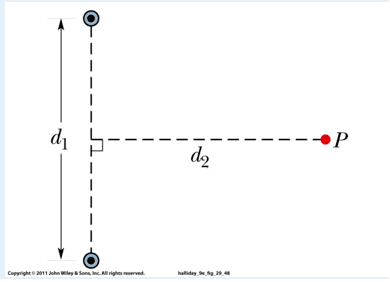# Biot Savart Law Problem

## Homework Statement

The figure shows two very long straight wires (in cross section) that each carry a current of 3.19 A directly out of the page. Distance d1 = 6.00 m and distance d2 = 4.00 m. What is the magnitude of the net magnetic field at point P, which lies on a perpendicular bisector to the wires?## Homework Equations

Biot-Savart's Law

## The Attempt at a Solution

${ B }_{ long,\quad straight\quad wire }\quad =\quad \frac { { \mu }_{ 0 }I }{ 2\pi r } \\ r\quad =\quad \sqrt { { (\frac { { d }_{ 1 } }{ 2 } ) }^{ 2 }\quad +\quad { { d }_{ 2 } }^{ 2 } } \\ { B }_{ total }\quad =\quad 2B\quad =\quad \frac { { \mu }_{ 0 }I }{ \pi r } \\ { B }_{ total }\quad =\quad 1.7695e-07$

I'm aware that I'm missing a sin(theta) in my multiplication, but why would I need to only specify a vertical component? The magnitude isn't only the vertical component.

TSny
Homework Helper
Gold Member
What is the direction of B for each current?

What is the direction of B for each current?
Both act upwards on the point

TSny
Homework Helper
Gold Member
The two fields at P are not in the same direction.

The two fields at P are not in the same direction.
Is this what you're visualizing:
Upper field points in the positive x and y direction
Lower field points in the negative x but positive y direction
?

TSny
Homework Helper
Gold Member
Yes.

Yes.
Alright that makes sense now to put the y component in. I was visualizing both fields pointing up at the same time, but that's not possible at all with the orientation of the field.

TSny
Homework Helper
Gold Member
OK.